# SAT Function Transformations: The Definitive Guide

The SAT will sometimes throw in a question on function transformations. This question can range from easy to difficult, so it's important to understand the entire concept. For example, take a look at the following official example taken from The College Board's Question of the Day app:

#### Example Function Tranformation SAT Question

The graph of the function $$f$$ is the graph of the function $$g$$ compressed horizontally by a factor of 2 and reflected over the $$y$$-axis. Which of the following correctly defines the function $$f$$?

A) $$f(x) = g(-2x)$$
B) $$f(x) = -g(2x)$$
C) $$f(x) = 2g(-x)$$
D) $$f(x) = -2g(x)$$

Before we get to the solution, let's review the transformations you need to know using our own example function

$f(x) = x^2 + 2x$

whose graph looks like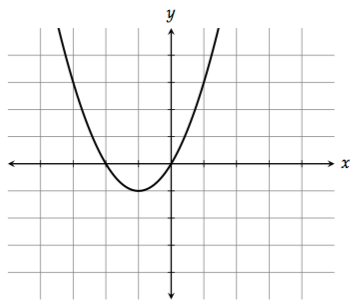#### 1. Shifting up and down

To shift the graph up, add a constant at the end of the function. For example, $$f(x) + 2 = x^2 + 2x + 2$$ would shift the graph up 2 units.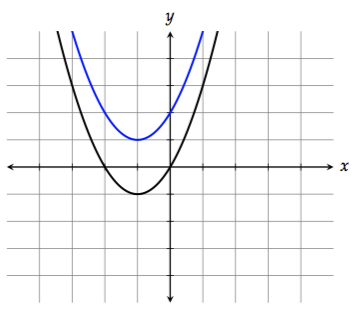To shift the graph down, subtract a constant at the end of the function. $$f(x) - 2 = x^2 + 2x - 2$$ would shift the graph down 2 units.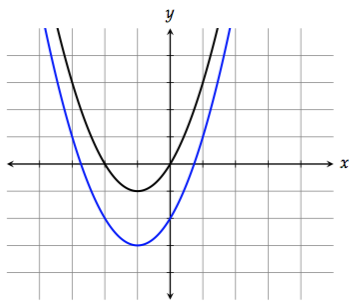#### 2. Shifting left and right

To shift the graph to the left by 1 unit, replace $$x$$ with $$x + 1$$:

$f(x + 1) = (x + 1)^2 + 2(x + 1)$To shift the graph to the right by 1 unit, replace $$x$$ with $$x - 1$$:

$f(x - 1) = (x - 1)^2 + 2(x - 1)$

The substitutions for left and right are counterintuitive to a lot of students because they're the opposite of what you might expect.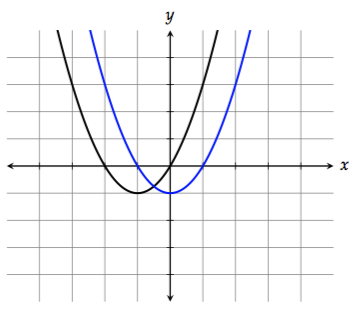#### 3. Reflecting across the $$x$$-axis

To reflect the graph across the $$x$$-axis (flip it upside down), multiply the function by $$-1$$:

$-f(x) = -x^2 - 2x$#### 4. Reflecting across the $$y$$-axis

To reflect the graph across the $$y$$-axis, replace $$x$$ with $$-x$$:

$f(-x) = (-x)^2 + 2(-x) = x^2 - 2x$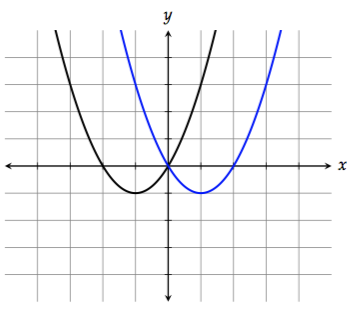#### 5. Stretching/Compressing vertically

To stretch the graph vertically by a factor of 2, multiply $$f(x)$$ by 2:

$2f(x) = 2(x^2 + 2x) = 2x^2 + 4x$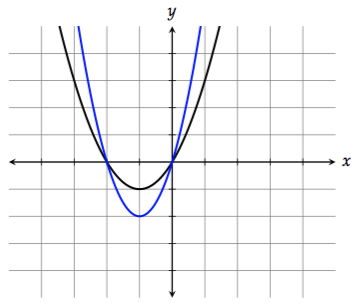You can think of this as the graph getting "taller."

To compress the graph vertically by a factor of 2, multiply $$f(x)$$ by $$\dfrac{1}{2}$$:

$\dfrac{1}{2}f(x) = \dfrac{1}{2}(x^2 + 2x) = \dfrac{1}{2}x^2 + x$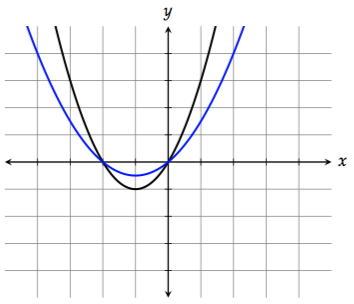Think of this as the graph getting "shorter."

#### 6. Stretching/Compressing horizontally

To stretch the graph horizontally by a factor of 2, replace $$x$$ with $$\dfrac{1}{2}x$$:

$f\left(\dfrac{1}{2}x\right) = \left(\dfrac{1}{2}x\right)^2 + 2\left(\dfrac{1}{2}x\right) = \dfrac{1}{4}x^2 + x$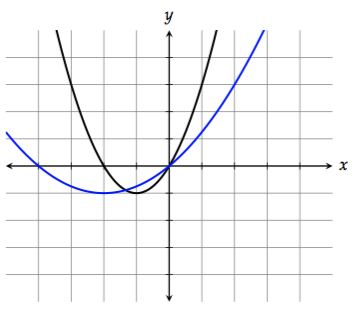Notice that the right side doesn't get stretched as much. That's because the points on the right side of the graph have smaller $$x$$-values (absolute value-wise). Doubling a small number doesn't do as much as doubling a large number.

To compress the graph horizontally by a factor of 2, replace $$x$$ with $$2x$$:

$f(2x) = (2x)^2 + 2(2x) = 4x^2 + 4x$

By the way, these substitutions for horizontal stretch and compression are also counterintuitive to a lot of students. Be careful of horizontal transformations (shifting left and right, stretching/compressing). It's often the opposite of what you might expect.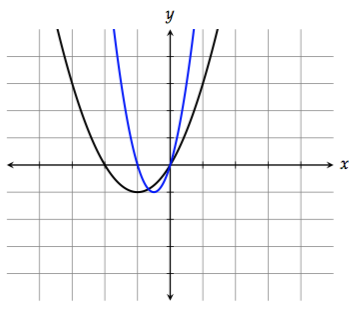Notice that the right side doesn't get compressed as much. That's because the points on the right side of the graph have smaller $$x$$-values (absolute value-wise). Halving a small number doesn't do as much as halving a large number.

#### 7. Absolute Value

To reflect all the points on the graph with negative $$y$$-values across the $$x$$-axis, take the absolute value of the function:

$\left|f(x)\right| = \left|x^2 + 2x\right|$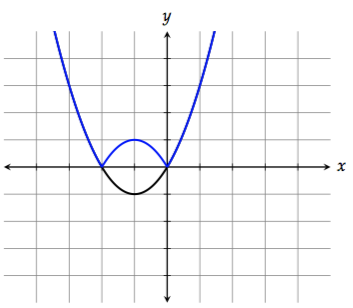#### Back to the Example

Getting back to the example question at the beginning of this post, we want to compress $$g$$ horizontally by a factor of 2 and reflect it over the $$y$$-axis. Given what we've learned, this is pretty easy.

To compress the graph horizontally by a factor of 2, we replace $$x$$ with $$2x$$:

$g(2x)$

To reflect the graph over the $$y$$-axis, we replace $$x$$ with $$-x$$:

$g(2(-x)) = g(-2x)$